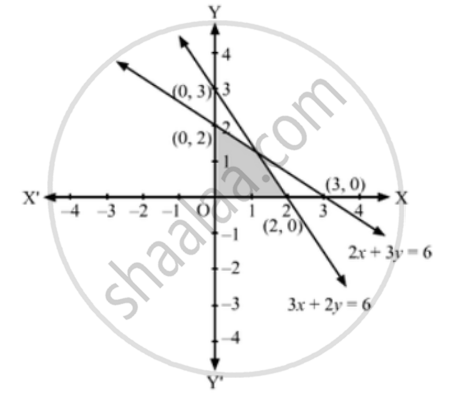Department of Pre-University Education, KarnatakaPUC Karnataka Science Class 11
Advertisement Remove all ads

# Solve the Following Systems of Linear Inequation Graphically: 2x + 3y ≤ 6, 3x + 2y ≤ 6, X ≥ 0, Y ≥ 0 - Mathematics

Solve the following systems of linear inequation graphically:

2x + 3y ≤ 6, 3x + 2y ≤ 6, x ≥ 0, y ≥ 0

Advertisement Remove all ads

#### Solution

Converting the inequations to equations, we obtain:
2x + 3y = 6, 3x + 2y = 6, x = 0, y = 0

2x + 3y =6:  This line meets the x-axis at (3,0) and the y-axis at (0, 2). Draw a thick line joining these points.
We see that the origin (0, 0) satisfies the inequation 2x + 3y ≤ 6
So, the portion containing  the origin represents the solution set of the inequation 2x + 3y ≤ 6

3x+2y =6:  This line meets the x-axis at (2, 0) and the y-axis at (0, 3). Draw a thick line joining these points.
We see that the origin (0,0) satisfies the inequation 3x + 2y ≤ 6.
So, the portion containing  the origin represents the solution set of the inequation 3x + 2y ≤ 6

Clearly, x ≥ 0, y ≥ 0 represents the first quadrant.
Hence. the shaded region in the figure represents the solution set of the given set of inequations.Is there an error in this question or solution?
Advertisement Remove all ads

#### APPEARS IN

RD Sharma Class 11 Mathematics Textbook
Chapter 15 Linear Inequations
Exercise 15.6 | Q 1.1 | Page 30
Advertisement Remove all ads

#### Video TutorialsVIEW ALL 

Advertisement Remove all ads
Share
Notifications

View all notifications

Forgot password?